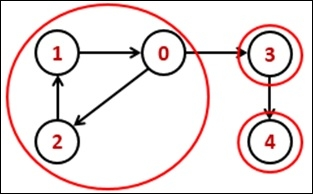# Strongly Connected Graphs

In a directed graph is said to be strongly connected, when there is a path between each pair of vertices in one component.To solve this algorithm, firstly, DFS algorithm is used to get the finish time of each vertex, now find the finish time of the transposed graph, then the vertices are sorted in descending order by topological sort.

## Input and Output

Input:
0 0 1 1 0
1 0 0 0 0
0 1 0 0 0
0 0 0 0 1
0 0 0 0 0

Output:
Following are strongly connected components in given graph:
0 1 2
3
4

## Algorithm

traverse(graph, start, visited)

Input: The graph which will be traversed, the starting vertex, and flags of visited nodes.

Output: Go through each node in the DFS technique and display nodes.

Begin
mark start as visited
for all vertices v connected with start, do
if v is not visited, then
traverse(graph, v, visited)
done
End

topoSort(u, visited, stack)

Input − The start node, flag for visited vertices, stack.

Output − Fill stack while sorting the graph.

Begin
mark u as visited
for all node v, connected with u, do
if v is not visited, then
topoSort(v, visited, stack)
done
push u into the stack
End

getStrongConComponents(graph)

Input: The given graph.

Output − All strongly connected components.

Begin
initially all nodes are unvisited
for all vertex i in the graph, do
if i is not visited, then
topoSort(i, vis, stack)
done

make all nodes unvisited again
transGraph := transpose of given graph

while stack is not empty, do
pop node from stack and take into v
if v is not visited, then
traverse(transGraph, v, visited)
done
End

## Example

#include <iostream>
#include <stack>
#define NODE 5
using namespace std;

int graph[NODE][NODE] = {
{0, 0, 1, 1, 0},
{1, 0, 0, 0, 0},
{0, 1, 0, 0, 0},
{0, 0, 0, 0, 1},
{0, 0, 0, 0, 0}
};

int transGraph[NODE][NODE];

void transpose() {    //transpose the graph and store to transGraph
for(int i = 0; i<NODE; i++)
for(int j = 0; j<NODE; j++)
transGraph[i][j] = graph[j][i];
}

void traverse(int g[NODE][NODE], int u, bool visited[]) {
visited[u] = true;    //mark v as visited
cout << u << " ";

for(int v = 0; v<NODE; v++) {
if(g[u][v]) {
if(!visited[v])
traverse(g, v, visited);
}
}
}

void topoSort(int u, bool visited[], stack<int>&stk) {
visited[u] = true;    //set as the node v is visited

for(int v = 0; v<NODE; v++) {
if(graph[u][v]) {    //for allvertices v adjacent to u
if(!visited[v])
topoSort(v, visited, stk);
}
}

stk.push(u);    //push starting vertex into the stack
}

void getStrongConComponents() {
stack<int> stk;
bool vis[NODE];

for(int i = 0; i<NODE; i++)
vis[i] = false;    //initially all nodes are unvisited

for(int i = 0; i<NODE; i++)
if(!vis[i])    //when node is not visited
topoSort(i, vis, stk);

for(int i = 0; i<NODE; i++)
vis[i] = false;    //make all nodes are unvisited for traversal
transpose();    //make reversed graph

while(!stk.empty()) {    //when stack contains element, process in topological order
int v = stk.top(); stk.pop();
if(!vis[v]) {
traverse(transGraph, v, vis);
cout << endl;
}
}
}

int main() {
cout << "Following are strongly connected components in given graph: "<<endl;
getStrongConComponents();
}

## Output

Following are strongly connected components in given graph:
0 1 2
3
4